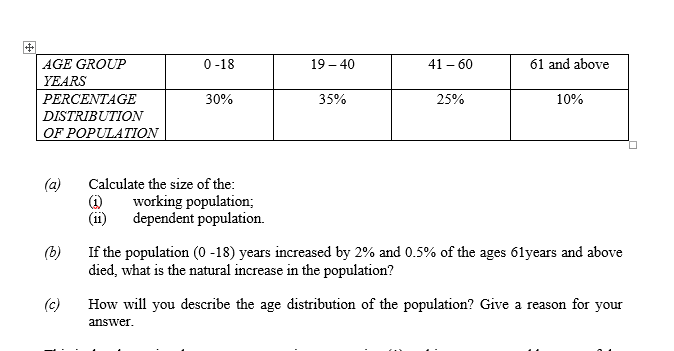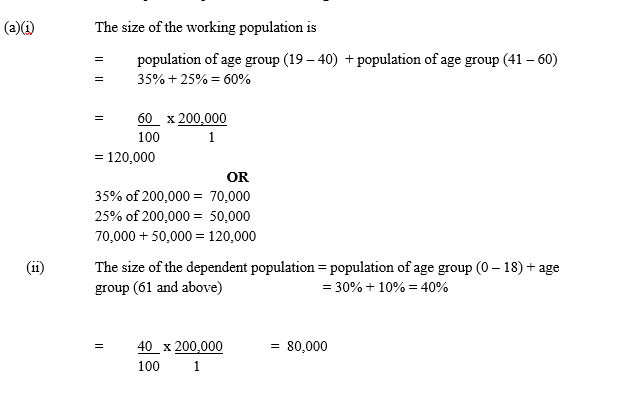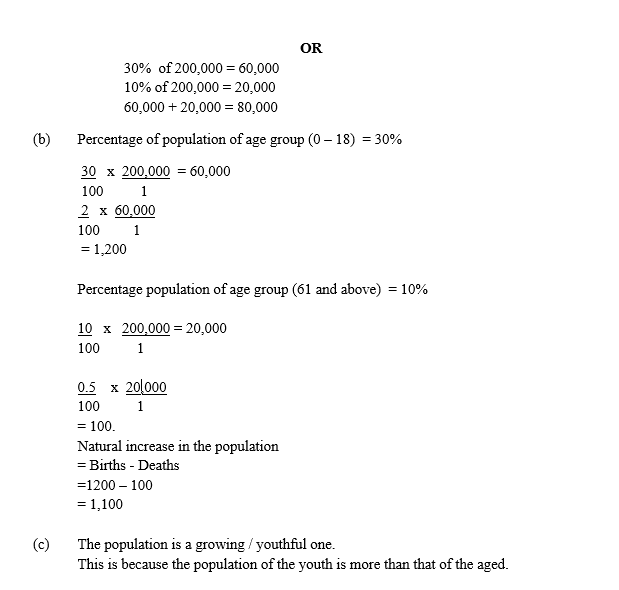WASSCE FOR PRIVATE CANDIDATES 2019 FIRST SERIES PAPER 2

QUESTION 2

The table below shows the percentage age distribution of population of country Y in 2010. The population was 200,000. Use the information in the table to answer the questions that follow.Observation

This is the alternative data response question to question (1) and it was attempted by most of the candidates. The question required candidates to calculate the working and dependent population, the natural growth rate and describe the age distribution of the population in the (a), (b) and (c) parts of the question respectively. Most of the candidates who attempted this question were able to calculate the working and dependent population in the (a) part of the question but only few of them could describe the age distribution of the population in the (c) part of the question. Candidates performed was slightly above average in this question.

The candidates were expected to provide the following answers to obtain the maximum marks.